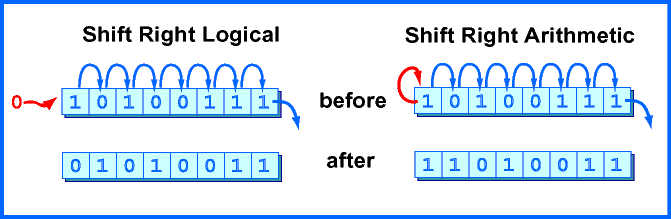```1111 1111 1111 0000  →  0011 1111 1111 1100
```

Is the resulting pattern the correct representation for -16/4? No. The result represents a large positive number, not -4

# Shift Right ArithmeticA right shift logical can not be used to divide a negative integer by two. The problem is that a shift right logical moves zeros into the high order bit. This is desirable in some situations, but not for dividing negative integers where the high order bit is the "sign bit."

An arithmetic right shift replicates the sign bit as needed to fill bit positions. The "sign bit" is the high order bit of the pattern. It can be a zero or a one. Whatever it is, it is replicated as needed to fill the positions that have been moved to the right.

Of course, on MIPS, the full 32-bit pattern in a register is shifted and the sign bit is bit 31 of that pattern.

### QUESTION 13:

Is there a need for an arithmetic shift left instruction?